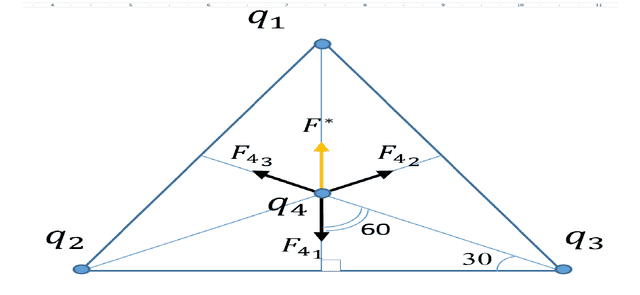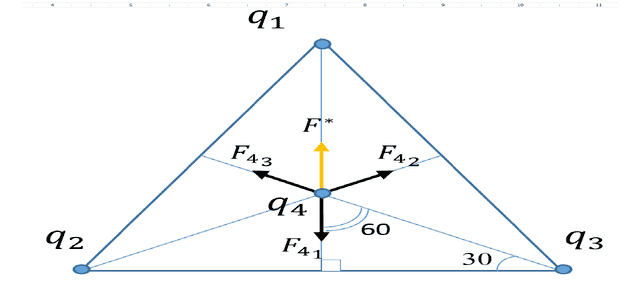# Charged particle in centre of equilateral triangle

• prehisto

## Homework Statement

3 charged particles located at equilateral triangles corners has charge ##q_1=q_2=q_2=4*10^{-6} C ## The 4th particle with charge ## q_4=3*10^{-4}## is placed at bisectors crossing. The distance between q1 and q4 is 3.46 cm .What is the net force on q4?## The Attempt at a Solution

So I calculated the forces ## F_{43} =F_{42}=F_{41}= (k q_1 q_2 )/ r^2 =9kN ##
Next I thought I calculate the F* and sum of ## F_{41} and F* ##
##F*=F^*_{43} +F^*_{42}=F_{43}/cos(60)+F_{42}/cos(60)= 36kN##

Now when i add up ##F* +F_{41}=27kN## Its getting confusing , because I was certain that sum of all forces on q4 should be zero,because of the symmetry and q1=q2=q3.

My question to you guys is, is my calculation wrong or the sum of all forces should not be zero?

## Homework Statement

3 charged particles located at equilateral triangles corners has charge ##q_1=q_2=q_2=4*10^{-6} C ## The 4th particle with charge ## q_4=3*10^{-4}## is placed at bisectors crossing. The distance between q1 and q4 is 3.46 cm .What is the net force on q4?## The Attempt at a Solution

So I calculated the forces ## F_{43} =F_{42}=F_{41}= (k q_1 q_2 )/ r^2 =9kN ##
Next I thought I calculate the F* and sum of ## F_{41} and F* ##
##F*=F^*_{43} +F^*_{42}=F_{43}/cos(60)+F_{42}/cos(60)= 36kN##

Now when i add up ##F* +F_{41}=27kN## Its getting confusing , because I was certain that sum of all forces on q4 should be zero,because of the symmetry and q1=q2=q3.

My question to you guys is, is my calculation wrong or the sum of all forces should not be zero?
I think the sum should be zero, but you need to show the vector sum of the vector forces to show it. Use rectangular coordinates to write the equations...Use rectangular coordinates to write the equations...Thank you for responding.

But i think I am did exactly that. If we imagine that direction from q4 to q1 ir positive axis direction . I projected two vectors to x-axis and added them up, then subtracted the third.

Thank you for responding.

But i think I am did exactly that. If we imagine that direction from q4 to q1 ir positive axis direction . I projected two vectors to x-axis and added them up, then subtracted the third.
I guess the way you wrote it is not intuitive for me, and doesn't appear to show the forces in both the x and y directions. I was looking for something more like this:

ΣFx = Fx14 + Fx24 + Fx34

ΣFy = Fy14 + Fy24 + Fy34

Can you express your work more in that format?Can you express your work more in that format?##Fx=Fx_{14} +Fx_{24}+Fx_{34}=F_{14}/cos(60)+F_{24}/cos(60)+Fx_{34}= 18+18-9=27kN##
##Fy=Fy_{14} +Fy_{24}+Fy_{34}=F_{14}*cos(90)+F_{24}*cos(30)+F_{34}*cos(30)= 0+7,24+7,24=15,48kN##
looking at the final values,looks like something is terribly wrong , but i don't see it :(

##Fx=Fx_{14} +Fx_{24}+Fx_{34}=F_{14}/cos(60)+F_{24}/cos(60)+Fx_{34}= 18+18-9=27kN##
##Fy=Fy_{14} +Fy_{24}+Fy_{34}=F_{14}*cos(90)+F_{24}*cos(30)+F_{34}*cos(30)= 0+7,24+7,24=15,48kN##
looking at the final values,looks like something is terribly wrong , but i don't see it :(
In the x-direction, Fx14 is zero, right? And Fx24 and Fx34 should be the same, differing only by their signs... And so on...

n the x-direction, Fx14 is zero, right? And Fx24 and Fx34 should be the same, differing only by their signs... And so on...

jess! I completely messed up there, i need to get some sleep :D.
Thank you!